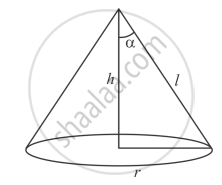Advertisement Remove all ads

# The Radius of the Base of a Right Circular Cone of Semi-vertical Angle α is R. Show that Its Volume is 1 3 π R 3 Cot α and Curved Surface Area is πR2 Cosec α. - Mathematics

Answer in Brief

The radius of the base of a right circular cone of semi-vertical angle α is r. Show that its volume is $\frac{1}{3} \pi r^3$ cot α and curved surface area is πr2 cosec α.

Advertisement Remove all ads

#### Solution$\sin \alpha = \frac{r}{l}$

$\Rightarrow r \cos ec \ \alpha = l$

$\tan \alpha = \frac{r}{h}$

$\Rightarrow\text { r cot } \alpha = h$

"volume = 1/3 pir^2h"

=1/3 pir^2 . r cost \ alpha

=1/3 pir^2 cot \ alpha

Surface area = pirl

= πr2 cosec α.

= πr2 cosec α.

Is there an error in this question or solution?
Advertisement Remove all ads

#### APPEARS IN

RD Sharma Class 10 Maths
Chapter 14 Surface Areas and Volumes
Q 39 | Page 83
Advertisement Remove all ads
Advertisement Remove all ads
Share
Notifications

View all notifications

Forgot password?